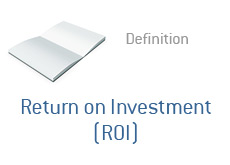## Definition of Return on Investment (ROI)

What does Return on Investment, or ROI, mean? What is the definition of Return on Investment?

Will an investment (of any kind) yield positive returns? And if so, how much?

To answer these questions, you need to calculate the ROI (Return on Investment).The simplest way to explain Return on Investment is to present you with the formula that is used to calculate ROI:

(Return From Investment - Initial Cost of Investment) / Initial Cost of Investment

So, let's say that you invested \$10,000 into a venture and ended up receiving \$15,000 back (your initial \$10,000 investment, plus \$5,000 in profit).

To figure out your ROI, simply substitute these values into the equation given above:

(\$15,000 - \$10,000) / \$10,000

\$5,000 / \$10,000 = .5

This would mean that you saw a ROI of 50%, which would be a "positive return on investment."

Let's say that you ended up receiving just \$7,500 of your original \$10,000 investment back.

(\$7,500 - \$10,000) / \$10,000

-\$2,500 / \$10,000 = -.25

This would mean that you saw a ROI of -25%, which would be a "negative return on investment".

This is the simplest definition of the term "Return on Investment".

--

Davemanuel.com Articles That Mention Return on Investment (ROI):

None